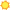15-2041.01 BiostatisticiansBright Outlook   2 closely related tasks Apply research or simulation results to extend biological theory or recommend new research projects. Develop or use mathematical models to track changes in biological phenomena, such as the spread of infectious diseases. 15-2031.00 Operations Research Analysts2 closely related tasks Define data requirements and gather and validate information, applying judgment and statistical tests. Break systems into their components, assign numerical values to each component, and examine the mathematical relationships between them. 15-2021.00 Mathematicians Apply mathematical theories and techniques to the solution of practical problems in business, engineering, the sciences, or other fields. 15-2041.00 StatisticiansAdapt statistical methods to solve specific problems in many fields, such as economics, biology, and engineering. 15-2051.00 Data ScientistsPropose solutions in engineering, the sciences, and other fields using mathematical theories and techniques.